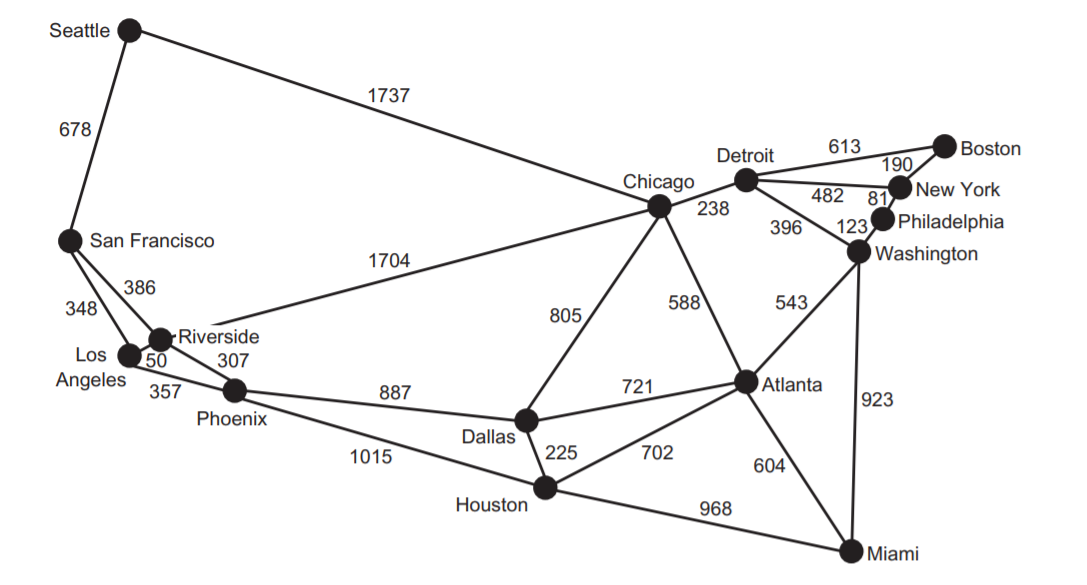# Traversing Graphs using Pytholog# Finding Paths in a Graph using Pytholog

We will use pytholog library.

We will define a weighted undirected graph for the largest MSAs in USA.

Image and examples source is “Classic Computer Science Problems in Python” book.

We first import the library and define the nodes and edges of the graph as prolog facts and rules.

```import pytholog as pl

graph_kb = pl.KnowledgeBase("MSA_graph")
"route(seattle, chicago, 1737)",
"route(seattle, san_francisco, 678)",
"route(san_francisco, riverside, 386)",
"route(san_francisco, los_angeles, 348)",
"route(los_angeles, riverside, 50)",
"route(los_angeles, phoenix, 357)",
"route(riverside, phoenix, 307)",
"route(riverside, chicago, 1704)",
"route(phoenix, dallas, 887)",
"route(phoenix, houston, 1015)",
"route(dallas, chicago, 805)",
"route(dallas, atlanta, 721)",
"route(dallas, houston, 225)",
"route(houston, atlanta, 702)",
"route(houston, miami, 968)",
"route(atlanta, chicago, 588)",
"route(atlanta, washington, 543)",
"route(atlanta, miami, 604)",
"route(miami, washington, 923)",
"route(chicago, detroit, 238)",
"route(detroit, boston, 613)",
"route(detroit, washington, 396)",
"route(detroit, new_york, 482)",
"route(boston, new_york, 190)",
## define the rules how can we move from one point to another
"path(X, Y, P) :- route(X, Y, P)",
"path(X, Y, P) :- route(X, Z, P2), path(Z, Y, P3), P is P2 + P3",
## to make it undirected (two-way) graph
#"path(X, Y, P) :- route(Y, X, P)",
"path(X, Y, P) :- route(Y, Z, P2), path(Z, X, P3), P is P2 + P3"
])```

We only needed to define the facts, the route between a city and its adjacent cities and the distance between them, then define the rule to traverse the graph searching for the path. Now let’s search for some paths between some cities.

pytholog uses Breadth-First Search algorithm to search for paths, So not always the result will be the shortest path but most of the cases it is. One more things the visited note returned when show_path = True, can be more than the one used to calculate the weight reponse, this is because the bfs search checks all nodes next to the current node and return it as visited, but you can see the actual path from the image which will lead to the result weight. Future implementations will support other kind of search algorithms.

```x, y = graph_kb.query(pl.Expr("path(boston, miami, Weight)"), cut = True, show_path = True)
## cut argument to stop searching when a path is found
print(x)
print([x for x in y if str(x) > "Z"]) ## remove weights in the visited nodes

# [{'Weight': 1317}]

The shortes possible path between the two cities!

###### N.B. The path given isn’t sorted.
```## the other way
x, y = graph_kb.query(pl.Expr("path(miami, boston, Weight)"), cut = True, show_path = True)
print(x)
[x for x in y if str(x) > "Z"]

# [{'Weight': 1317}]

x, y = graph_kb.query(pl.Expr("path(seattle, washington, Weight)"), cut = True, show_path = True)
print(x)
[x for x in y if str(x) > "Z"]

# [{'Weight': 2371}]
# ['chicago', 'detroit']

x, y = graph_kb.query(pl.Expr("path(san_francisco, atlanta, Weight)"), cut = True, show_path = True)
print(x)
[x for x in y if str(x) > "Z"]

# [{'Weight': 2678}]
# ['houston', 'dallas', 'riverside', 'chicago']```

Note here the weight is the second shortest path but it can be enhanced by better defining the facts and rules. Note also that the path given show “Houston & Dallas” but if you calculate the weights you will find that the algorithm never passed by them. It only passed to Riverside then Chicago then Atlanta. But the value is given because they were checked.

```x, y = graph_kb.query(pl.Expr("path(chicago, detroit, Weight)"), cut = True, show_path = True)
print(x)
[x for x in y if str(x) > "Z"]

# [{'Weight': '238'}]
# []

x, y = graph_kb.query(pl.Expr("path(los_angeles, dallas, Weight)"), cut = True, show_path = True)
print(x)
[x for x in y if str(x) > "Z"]

# [{'Weight': 1244}]
# ['phoenix']

x, y = graph_kb.query(pl.Expr("path(riverside, washington, Weight)"), cut = True, show_path = True)
print(x)
[x for x in y if str(x) > "Z"]

# [{'Weight': 2338}]
# ['miami', 'chicago', 'atlanta', 'detroit']```

The lowest weight was given but again “Miami” was returned although when calculating the weights we will find out that the algorithm never passed by it but it checked it because of the return rule defined.

As we saw, pytholog works great to calculate weights and distances between points in a weighted graph. While getting the path needs further improvements in newer versions of the library.